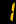# Teaching Strategy

MathConcept uses a distinctive combination of mental, verbal, visual, tactile, and written methods to help students grasp fundamental math concepts and regain confidence and self–esteem.

MathConcept also assists students to work in advance at all levels by providing enrichment topics not usually being taught in school.

## Mental

Students are taught how and when to use mental math techniques. This enables them to develop confidence and so they will be more willing to explore math, in addition speeding up the time required to solve various math problems.

`e.g. 99 × 3`

Instead of working through the problem traditionally by hand using vertical form, students are taught to think mentally as follow:

`99 = 100 − 1,⇒ 99 × 3 = 300 − 3 = 297.`

## Verbal

We ensure students are being taught in language that makes sense to them, by breaking down the meaning of root words in the mathematics context. Students are also trained to express their work and reasoning verbally.

`e.g. 12% of 300`

We first break down per-cent into for each group of 100. From this, we then guide the student to think "12% of 300" as "having 12 in every group of 100” and hence we have three groups, then

`12 + 12 + 12 = 36`

## Visual

Significant portion of MathConcept printed courseware contain meaningful pictures, charts, and tables to help explain different concepts. Offering students deeper insights into problems that transcend the written words.

`e.g. $\frac{3}{4}$ of 20`

Student can first think of 20 being broken into four equal parts,And now taking 3 parts gives us

`5 + 5 + 5 = 15`

## Tactile

Teaching tools are used to help introducing, explaining, and reinforcing concepts and skills. By actually doing the math themselves helps students to learn to analysis the problem, organize the logical steps, and present the final solution to the teacher.

## Written

MathConcept provides plentiful written practices with computation for the the systematic development of mathematical thought and skills. Our printed courseware covers the entire spectrum from practising number writing to more advanced mathematics such as calculus.e